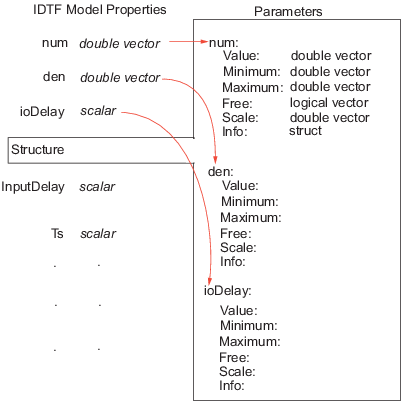Imposing Constraints on Model Parameter Values

All identified linear (IDLTI) models, except idfrd, contain a Structure property. The Structure property contains the adjustable entities (parameters) of the model. Each parameter has attributes such as value, minimum/maximum bounds, and free/fixed status that allow you to constrain them to desired values or a range of values during estimation. You use the Structure property to impose constraints on the values of various model parameters.

The Structure property contains the essential parameters that define the structure of a given model:

• For identified transfer functions, includes the numerator, denominator, and delay parameters

• For polynomial models, includes the list of active polynomials

• For state-space models, includes the list of state-space matrices

For information about other model types, see the model reference pages.

For example, the following example constructs an idtf model, specifying values for the Numerator and Denominator parameters:

num = [1 2];
den = [1 2 2];
sys = idtf(num,den)

You can update the value of the Numerator and Denominator properties after you create the object as follows:

new_den = [1 1 10];
sys.Denominator = new_den;

To fix the denominator to the value you specified (treat its coefficients as fixed parameters), use the Structure property of the object as follows:

sys.Structure.Denominator.Value = new_den;
sys.Structure.Denominator.Free = false(1,3);

For a transfer function model, the Numerator, Denominator, and IODelay model properties are simply pointers to the Value attribute of the corresponding parameter in the Structure property.Similar relationships exist for other model structures. For example, the A property of a state-space model contains the double value of the state matrix. It is an alias to the A parameter value stored in Structure.A.Value.

System Identification Toolbox Documentation평가판 신청